Question

QUESTION 4 For the circuit in the figure, 8m1 - 8m2 8ms - 833 MA/V, 8m3...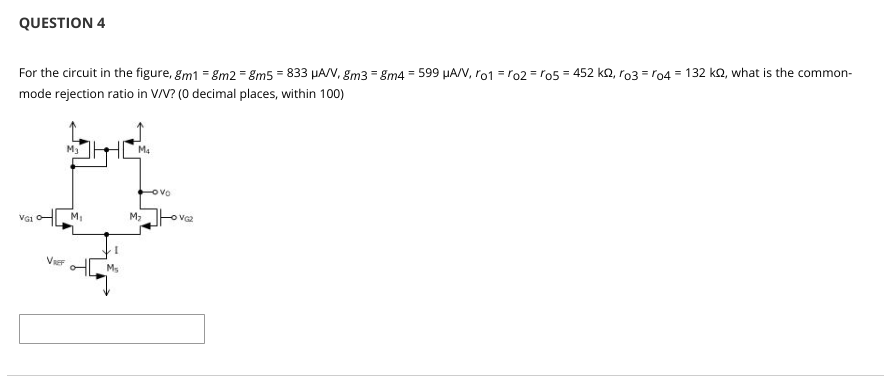QUESTION 4 For the circuit in the figure, 8m1 - 8m2 8ms - 833 MA/V, 8m3 = 8m4 = 599 JA/V, ro1 = ro2 = '05 - 452 kq, l'o3 = '04 = 132 k2, what is the common mode rejection ratio in V/V? (0 decimal places, within 100) MUM OVO VGM Move VE HIM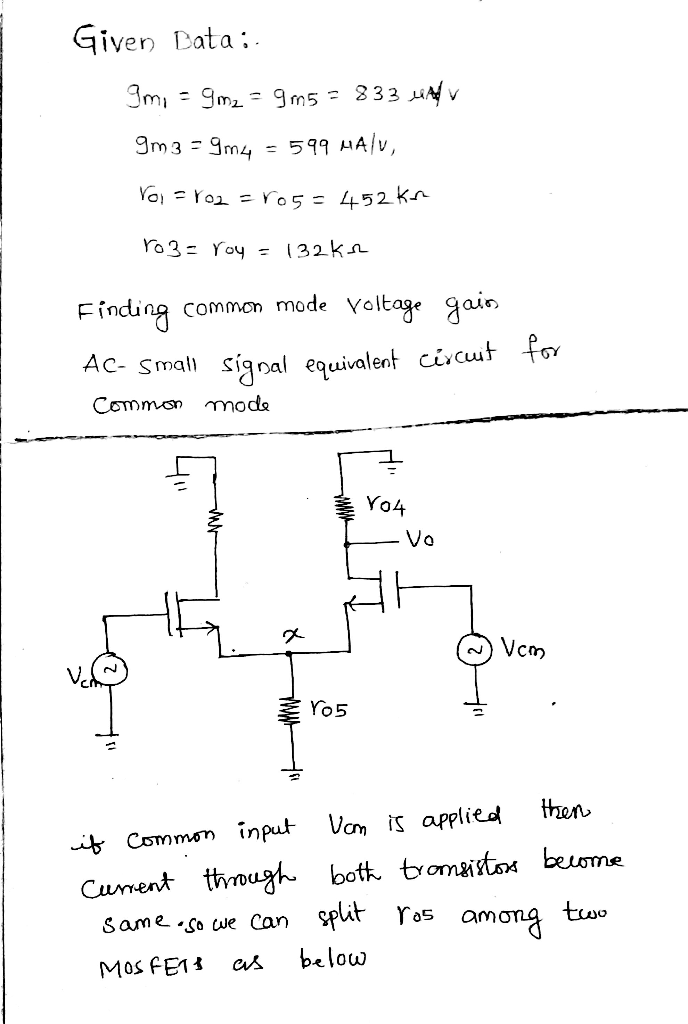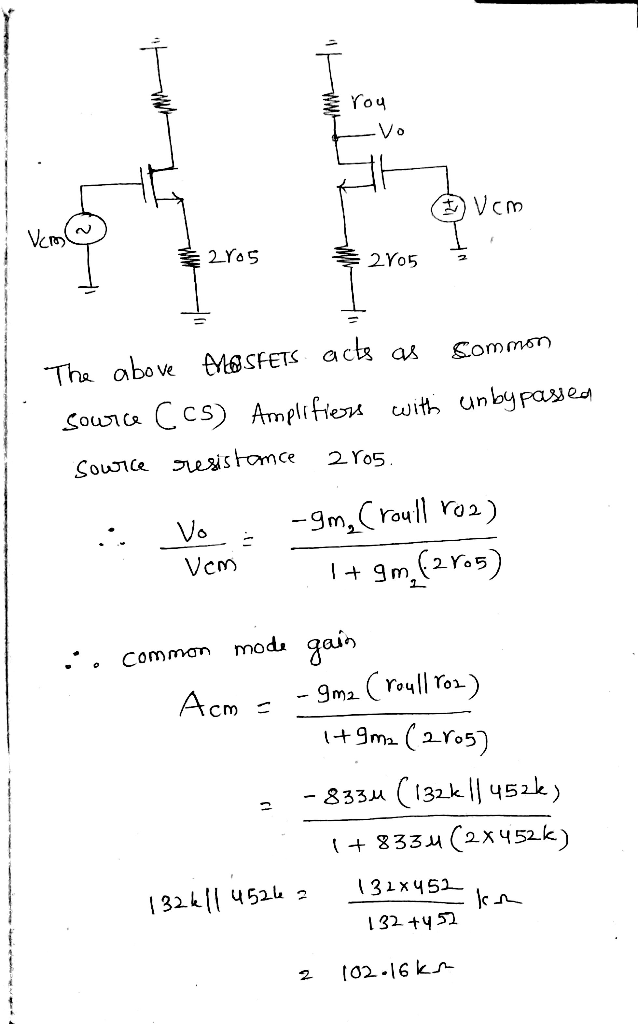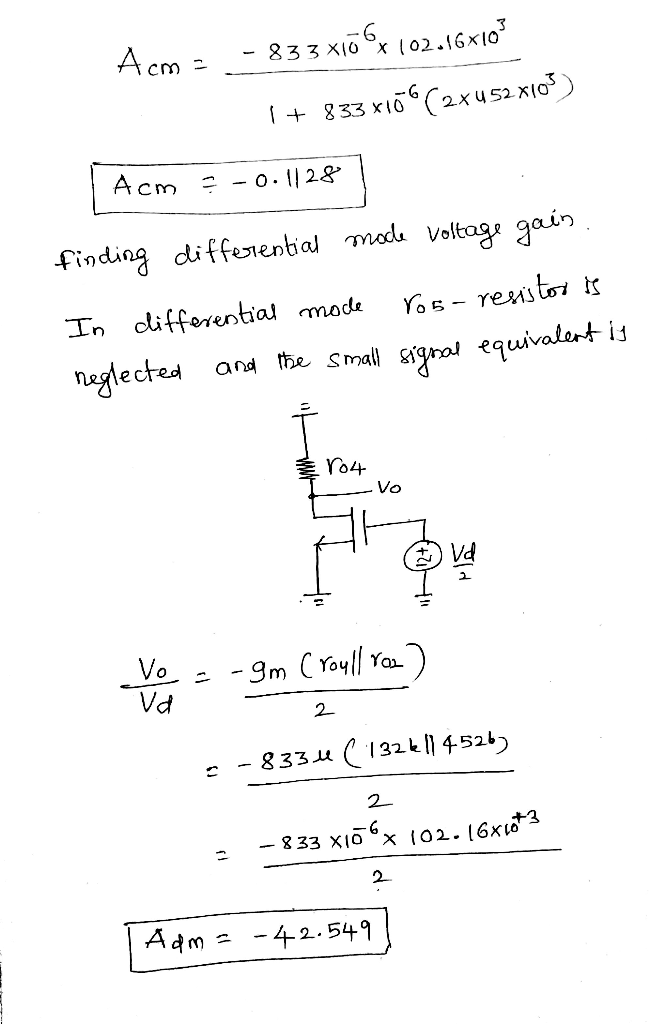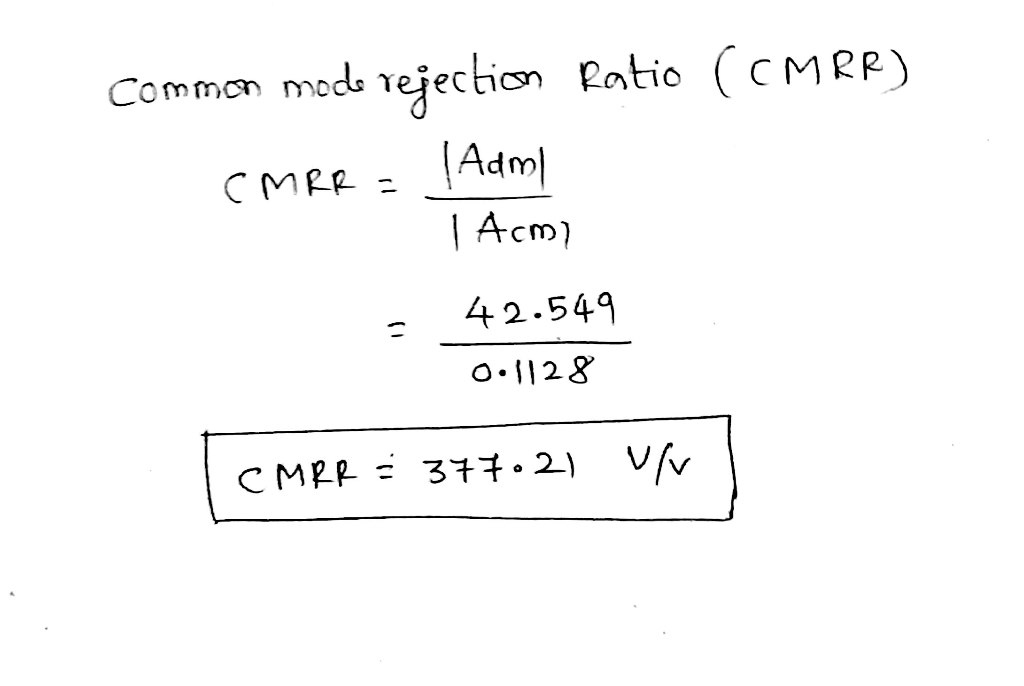Earn Coins

Coins can be redeemed for fabulous gifts.

Similar Homework Help Questions
• QUESTION 4 3 pc For the circuit in the figure, Em1 8m2 = 8m5 = 773...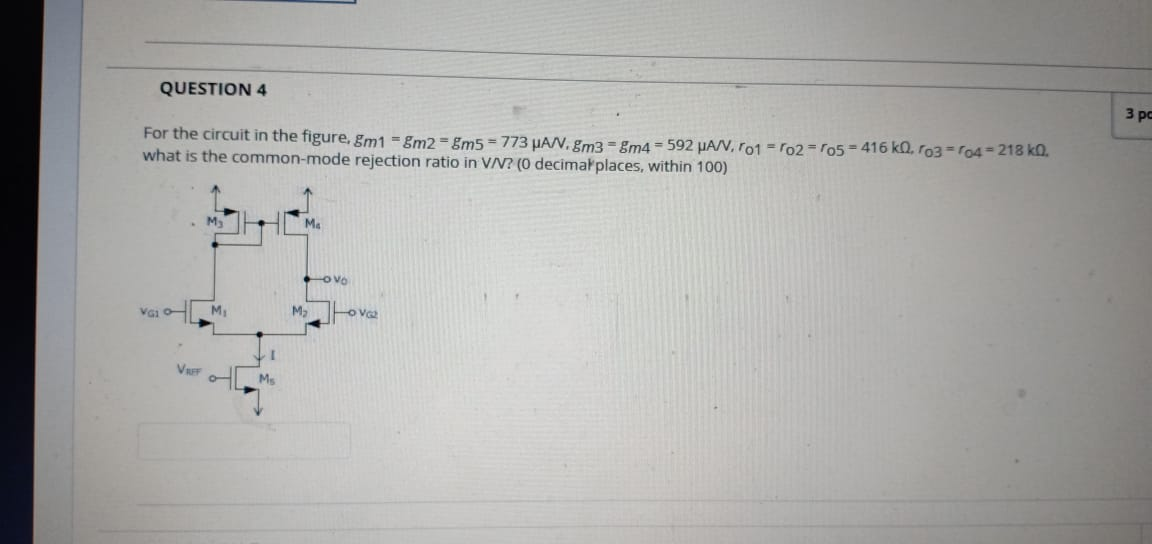QUESTION 4 3 pc For the circuit in the figure, Em1 8m2 = 8m5 = 773 MAX. m3 - m4 = 592 PA/V, T01 = '02-'05 - 416 ko. To3=104 = 218 ko. what is the common-mode rejection ratio in VN? (0 decimal places, within 100) MTH M

• Just question iv）plz n operational amplifier circuit is shown in Figure 3-1 +5 V 25 kQ...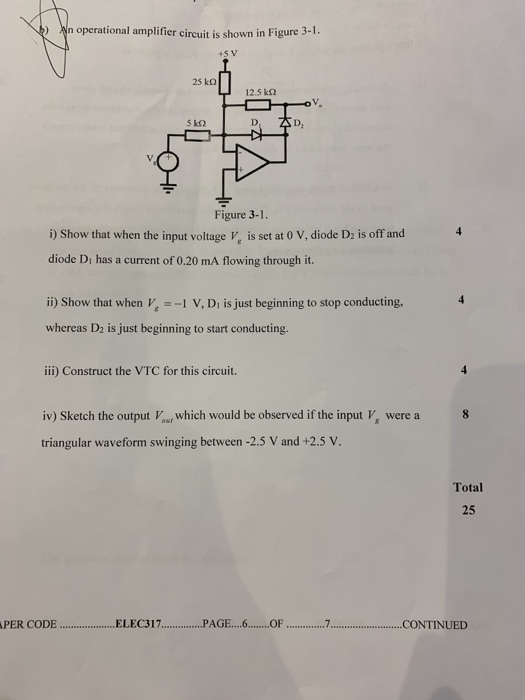Just question iv）plz n operational amplifier circuit is shown in Figure 3-1 +5 V 25 kQ 12.5 k2 5 kQ D, Figure 3-1. i) Show that when the input voltage V, is set at 0 V, diode Dz is off and diode Di has a current of 0.20 mA flowing through it. ii) Show that when v 1 V, Di is just beginning to stop conducting, whereas D2 is just beginning to start conducting. iii) Construct the VTC for this...

• A common source amplifier circuit based on a single n-channel MOSFET is shown in Figure 4b. Assume that the transconductance gm-60 mS (equivalent to mA/ V) and drain source resistance, os,...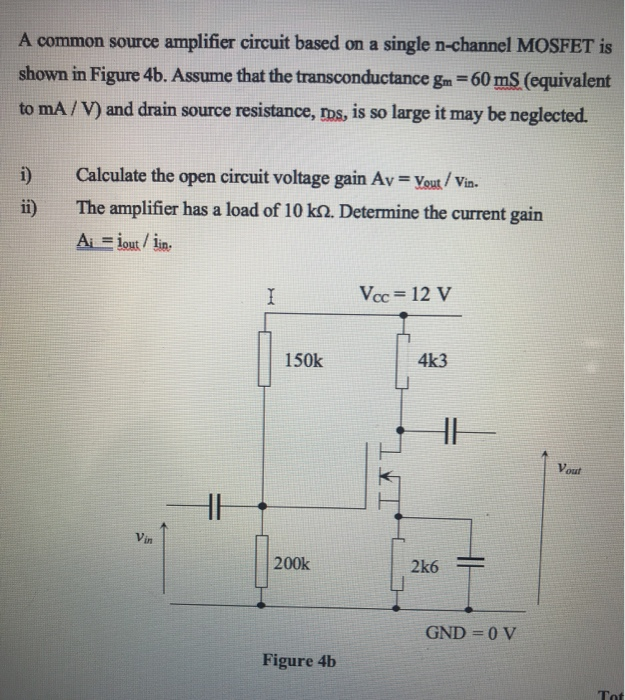A common source amplifier circuit based on a single n-channel MOSFET is shown in Figure 4b. Assume that the transconductance gm-60 mS (equivalent to mA/ V) and drain source resistance, os, is so large it may be neglected. 0) Calculate the open circuit voltage gain Av Yout/ Vis. i) The amplifier has a load of 10 k2. Determine the current gain Va. = 12 V 150k 4k3 Vout Vin 200k GND = 0 V Figure 4b a) State the name...